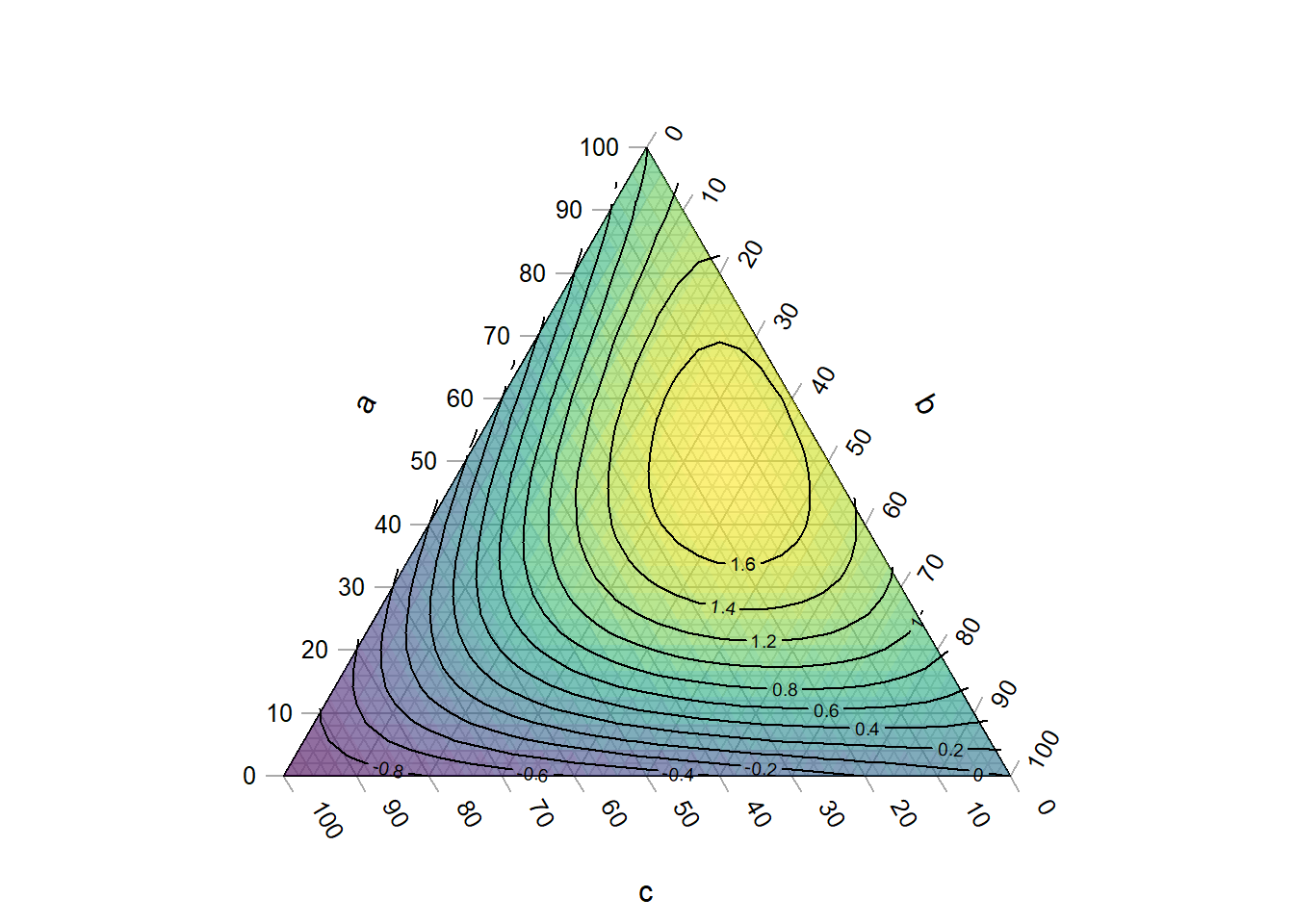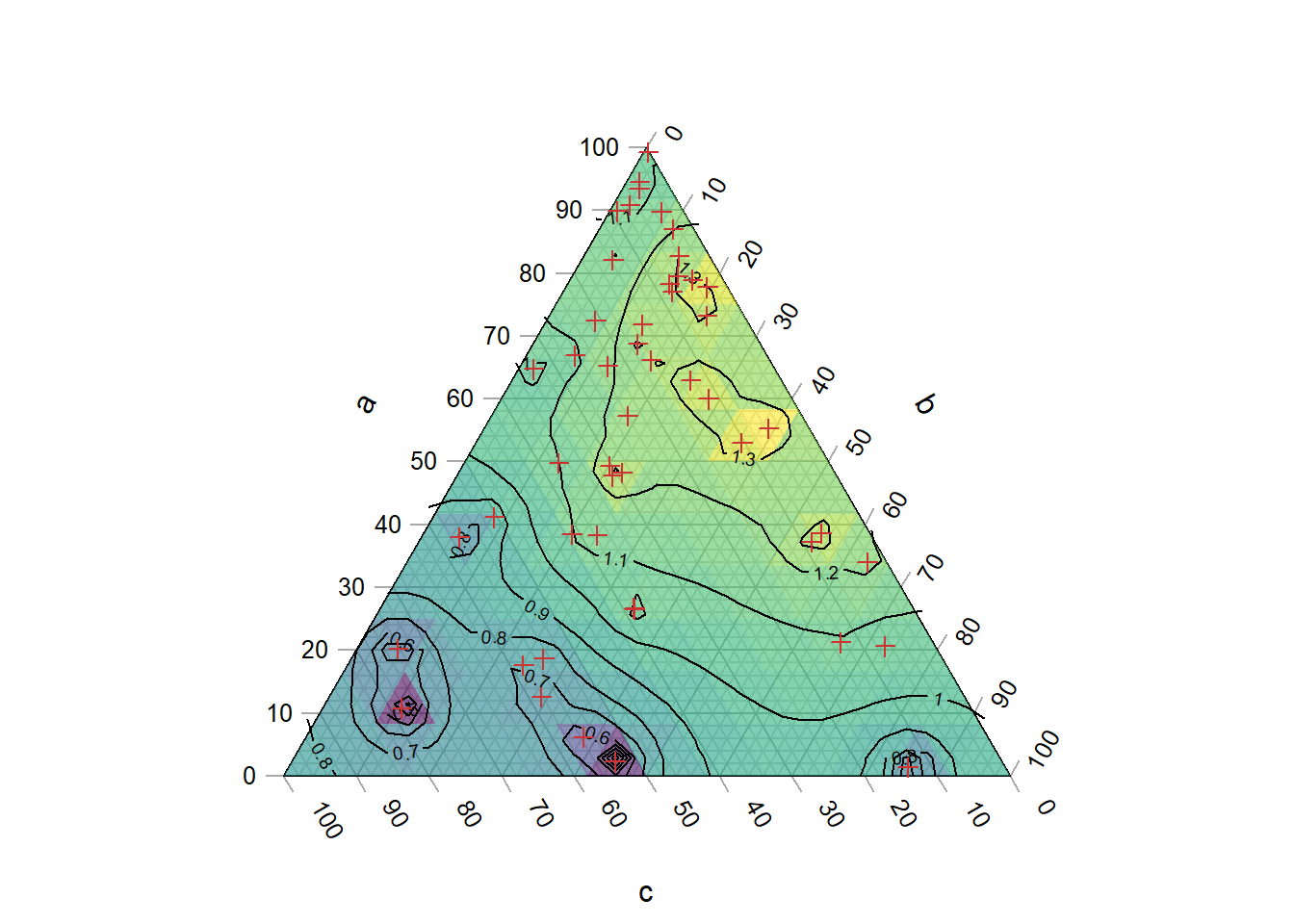A ternary plot can be shaded and contoured according to a known function:

library("Ternary")
par(mar = rep(0.2, 4))
TernaryPlot(alab = 'a', blab = 'b', clab = 'c')

FunctionToContour <- function (a, b, c) {
a - c + (4 * a * b) + (27 * a * b * c)
}

values <- TernaryPointValues(FunctionToContour, resolution = 24L)
ColourTernary(values)
TernaryContour(FunctionToContour, resolution = 36L)If it is not computationally feasible to execute a function at every point, it is possible to interpolate between known values.

Inverse distance weighting is a simple approach:

# Generate the value of a function at some random points
set.seed(0)
nPts <- 50
a <- runif(nPts)
b <- runif(nPts) * (1 - a)
c <- 1 - a - b
abc <- rbind(a, b, c)
response <- FunctionToContour(a, b, c)

# Start plot, to define coordinate system
par(mar = rep(0.2, 4))
TernaryPlot(alab = 'a', blab = 'b', clab = 'c')

# Convert measured points to XY
xy <- TernaryToXY(abc)

Predict <- function (predXY) {
Distance <- function (a, b) {
apply(a, 2, function (pt) sqrt(colSums((pt - b) ^ 2)))
}
dists <- Distance(xy, predXY)
id <- 1 / dists
idw <- id / rowSums(id)

# Return:
colSums(response * t(idw))
}

# Predict at triangle centres
tri <- TriangleCentres(resolution = 12L)
predicted <- Predict(tri[1:2, ])
map <- rbind(x = tri['x', ], y = tri['y', ], z = predicted,
down = tri['triDown', ])
ColourTernary(map)

# Calculate contours
PredictABC <- function (a, b, c) Predict(TernaryToXY(rbind(a, b, c)))
TernaryContour(PredictABC, resolution = 36L)

# Mark measured points
TernaryPoints(abc, pch = 3, col = '#cc3333')More sophisticated interpolation approaches are possible, e.g.  kriging; these may help to alleviate artefacts of sampling intensity as observed towards the bottom of the example plot above.